Name:    3rd Grade Basic Math Test 4

Multiple Choice
Identify the choice that best completes the statement or answers the question.

1.

Geometry – Congruency and Similarity – RIT 161 – 170
Which has congruent shapes?
 a.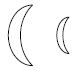d.b.e.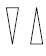c.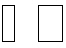2.

Geometry – Shapes and Figures – RIT 171 – 180
Which is a cylinder?
 a. shoebox d. soup can b. baseball e. book c. dice

3.

Geometry – Symmetry and Transformations – RIT 171 – 180
Which of these shows a flip?

 a.d.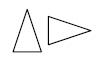b.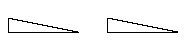e.c.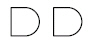4.

Geometry – Congruency and Similarity – RIT 171 – 180
Which shape is congruent to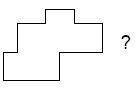a.d.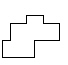b.e.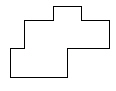c.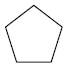5.

Geometry – Line Properties and Terminology – RIT 181 – 190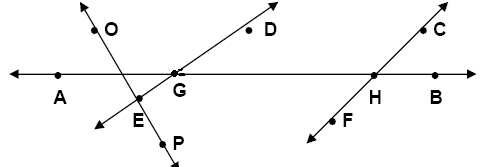Which point is on line DE?
 a. P d. G b. A e. H c. C

6.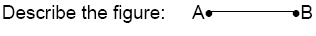a. Ray AB d.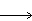AB b. Line AB e. Line Segment AB c.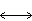AB

7.

Geometry – Shapes and Figures – RIT 181 – 190

Name these figures from left to right.a. circle, square, rectangle, triangle d. triangle, circle, square, rectangle b. square, rectangle, triangle, circle e. triangle, circle, rectangle, square c. rectangle, triangle, circle, square

8.

Which is a cube?
 a.d.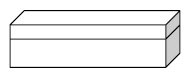b.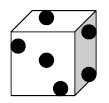e.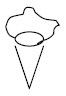c.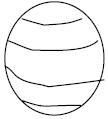9.

What flat shape has 3 sides and 3 corners?
 a. cube d. rectangle b. triangle e. sphere c. square

10.

Geometry – Symmetry and Transformations – RIT 181 – 190Which figures show a line of symmetry?
 a. 1, 4, and 5 d. 1 and 4 b. 2, 4, and 5 e. 2, 3, 4 c. 4 and 5

11.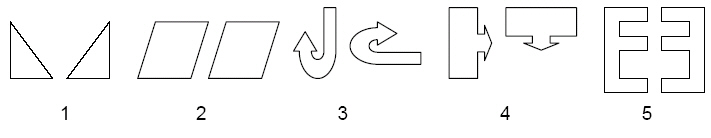Which pairs of objects have been turned?
 a. 1, 4, and 5 d. 2 and 3 b. 3 and 4 e. 3, 4, and 5 c. 1, 2, and 3

12.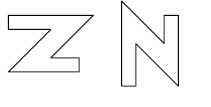The figure on the left has been moved to get the figure on the right. This is an
example of _____.
 a. symmetry d. congruency b. flip e. slide c. turn

13.

Geometry – Congruency and Similarity – RIT 181 – 190

Which angles are congruent?
 a.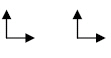c.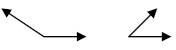b.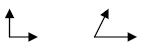d.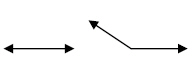14.

Which figures are similar, but not congruent?
 a.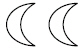d.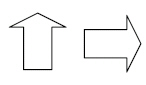b.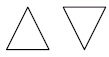e.c.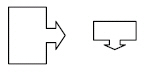15.

Measurement – Length, Height, Volume – RIT 151 – 160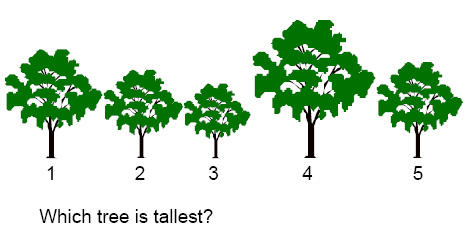a. 1 d. 4 b. 2 e. 5 c. 3

16.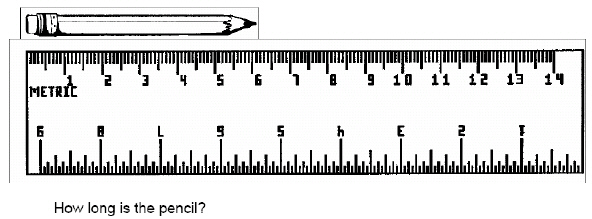a. 7 cm d. 5 cm b. 6 cm e. 3 cm c. 4 cm

17.

Measurement – Time, Temperature – RIT 151 – 160

What would you use to tell time?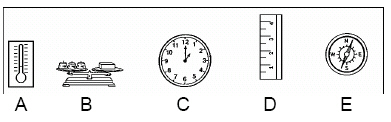a. A d. D b. B e. E c. C

18.

About what is the temperature on the thermometer?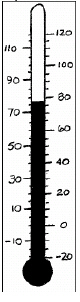a. 00 d. 800 b. 200 e. 500 c. 300

19.

Measurement – Money – RIT 161 – 170How much is the coin worth?
 a. 5 d. 50 b. 10 e. 25 c. 1

20.

Measurement – Time, Temperature – RIT 161 – 170

What month comes next?
January, February,
 a. October d. March b. December e. April c. September

21.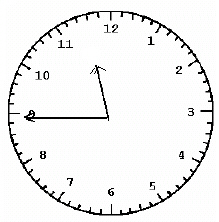What time is it on the clock?
 a. 8:58 d. 10:15 b. 12:09 e. 5:23 c. 11:44

22.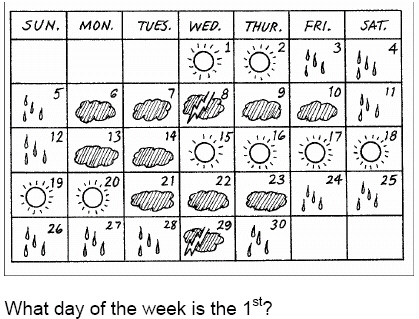a. Friday d. Wednesday b. Sunday e. Saturday c. Tuesday

23.What is the temperature in degrees Celsius?
 a. 800  C d. 600 C b. 1400 C e. 200  C c. 500  C